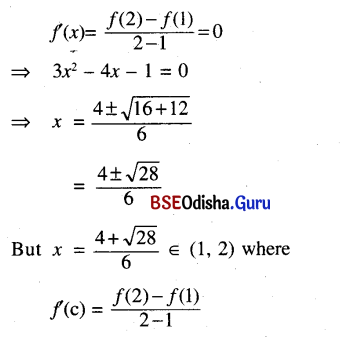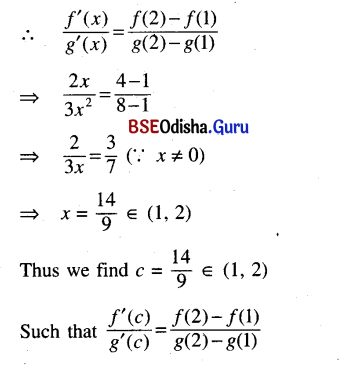# CHSE Odisha Class 12 Math Solutions Chapter 7 Continuity and Differentiability Ex 7(m)

Odisha State Board Elements of Mathematics Class 12 CHSE Odisha Solutions Chapter 7 Continuity and Differentiability Ex 7(m) Textbook Exercise Questions and Answers.

## CHSE Odisha Class 12 Math Solutions Chapter 7 Continuity and Differentiability Exercise 7(m)

Question 1.
Verify Rolle’s theorem for the function
f(x) = x (x – 2)2, 0 ≤ x ≤ 2.
Solution:
f(x) = x (x – 2)2, 0 ≤ x ≤ 2
Here a = 0, b = 2
f(x) is a polynomial function hence it is continuous and also differentiable.
∴ f is continuous on [0. 2]
f is differentiable on (0, 2)
f(0) = 0 = f(2)
Thus conditions of Rolle’s theorem are satisfied.
f'(x) = (x – 2)2 + 2x (x – 2)
= (x – 2) (x – 2 + 2x)
= (x – 2) (3x – 2)
f'(x)= 0 ⇒ x = 2, x = $$\frac{2}{3}$$
But x = 2 ∉ (0, 2). Thus c = $$\frac{2}{3}$$ such that f'(c) = 0
Thus Rolle’s theorem is verified.

Question 2.
Examine if Rolle’s theorem is applicable to the following functions:
(i) f(x) = |x| on [-1, 1]
Solution:
f(x) = |x| on [-1, 1]
As f(x) = |x| is not differentiable at x = 0 ∈ (-1, 1)
We have Rolle’s theorem is not applicable.

(ii) f(x) = [x] on [-1, 1]
Solution:
f(x) = [x] on [-1, 1]
f(x) = [x] is not continuous at 0 ∈ [-1, 1]
Rolle’s theorem is not applicable.

(iii) f(x) = sin x on [0, π]
Solution:
f(x) = sin x on [0, π]
f is a trigonometric function hence continuous and differentiable on its domain.
∴ f is continuous on [0, π]
f is differentiable on (0, π]
f(0) = f(π)
Thus Rolle’s theorem is applicable for f(x) = sin x on [0, π]

(iv) f(x) = cot x on [0, π]
Solution:
f(x) = cot x on [0, π]
Clearly cot (0) and cot (π] are not defined hence Rolle’s theorem is not applicable.Question 3.
Verify Lagrange’s Mean-Value theorem for
F(x) = x3 – 2x2 – x + 3 on [1, 2]
Solution:
f(x) = x3 – 2x2 – x + 3 on [1, 2]
f is a polynomial function hence continuous as well as differentiable.
∴ f is continuous on [1, 2]
f is differentiable on (1, 2)
Thus Largange’s mean value theorem is applicable.
Now f(1) = 1 – 2 – 1 + 3 = 1
f(2) = 8 – 8 – 2 + 3 = 1
∴ f(x) = 2x2 – 4x – 1Thus Lagrange’s mean value theorem is verified.

Question 4.
Test if Lagrange’s mean value theorem holds for the functions given in question no. 2.
Solution:
(i) f(x) = |x| is not differentiable at x = 0 ∈ (-1, 1)
Thus Lagrange’s mean value theorem, does not hold.

(ii) f(x) = [x] is discontinuous at 0 ∈ [-1, 1]
Thus Lagrange’s mean value theorem is not applicable.

(iii) f(x) = sin x is a trigonometric function, which is continuous as well as differentiable in its domain.
∴ f is continuous on [0, π]
f is differentiable on (0, π)
Thus conditions of Lagrange’s mean value theorem are satisfied.
Hence mean value theorem is applicable.

(iv) f(x) = cot x
Which is undefined x = 0 and x = π
Thus Lagrange’s mean value theorem is not applicable.Question 5.
(Not for examination) Verify Cauchy’s mean value theorem for the functions x2 and x3
in [1, 2].
Solution:
Let f(x) = x2, and g(x) = x3 on [1, 2]
Both f and g are polynomial functions, hence continuous and differentiable.
∴ f and g are continuous on [1, 2]
f and g are differentiable on (1, 2)
g'(x) = 3x2 ≠ 0 ∀ x ∈ (1, 2)
Thus conditions of Cauchy’s mean value theorem are satisfied.
Now f(1) = 1, f(2) = 4, g(1) = 1, g(2) = 8
f'(x) = 2, and g'(x) = 3x2